## Smoothing Splines

If your data is noisy, you might want to fit it using a smoothing spline. Alternatively, you can use one of the smoothing methods described in Filtering and Smoothing Data.

The smoothing spline s is constructed for the specified smoothing parameter p and the specified weights wi. The smoothing spline minimizes

`$p{\sum _{i}{w}_{i}{\left({y}_{i}-s\left({x}_{i}\right)\right)}^{2}+\left(1-p\right)\int \left(\frac{{d}^{2}s}{d{x}^{2}}\right)}^{2}dx$`

If the weights are not specified, they are assumed to be 1 for all data points.

p is defined between 0 and 1. p = 0 produces a least-squares straight-line fit to the data, while p = 1 produces a cubic spline interpolant. If you do not specify the smoothing parameter, it is automatically selected in the “interesting range.” The interesting range of p is often near 1/(1+h3/6) where h is the average spacing of the data points, and it is typically much smaller than the allowed range of the parameter. Because smoothing splines have an associated smoothing parameter, you might consider these fits to be parametric in that sense. However, smoothing splines are also piecewise polynomials like cubic spline or shape-preserving interpolants and are considered a nonparametric fit type in this guide.

Note

The smoothing spline algorithm is based on the `csaps` function.

The nuclear reaction data from the file carbon12alpha.mat is shown here with three smoothing spline fits. The default smoothing parameter (p = 0.99) produces the smoothest curve. The cubic spline curve (p = 1) goes through all the data points, but is not quite as smooth. The third curve (p = 0.95) misses the data by a wide margin and illustrates how small the “interesting range” of p can be.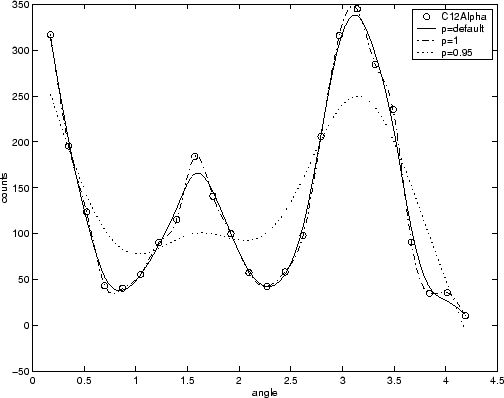### Selecting a Smoothing Spline Fit Interactively

In the Curve Fitting app, select `Smoothing Spline` from the model type list.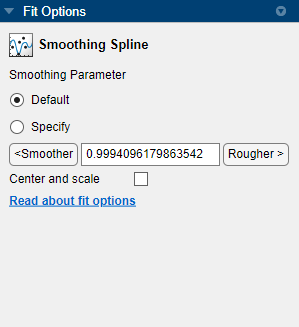You can specify the following options:

• To make a smoother fit further from the data, click the button repeatedly until the plot shows the smoothness you want.

• To make a rougher fit closer to the data, click the button until you are satisfied with the plot.

• Alternatively, specify any value from 0 to 1 for the smoothing parameter. 0 produces a linear polynomial fit (a least-squares straight-line fit to the data), while 1 produces a piecewise cubic polynomial fit that passes through all the data points (a cubic spline interpolant).

• Click Default to return to the initial value. The toolbox attempts to select a default value appropriate for your data. See About Smoothing Splines.

For example:

`load carbon12alpha`

2. In the Curve Fitting app, select `angle` for X data and `counts` for Y data.

3. Select the `Smoothing Spline` fit type.

4. Try smoothing parameter values `1`, `0.95`, and the default value (0.99).

### Fit Smoothing Spline Models Using the `fit` Function

This example shows how to use the `fit` function to fit a smoothing spline model to data.

Fit a Smoothing Spline Model

Load data and fit a smoothing spline model by specifying `'smoothingspline'` when calling the fit function.

```load enso f = fit(month,pressure,'smoothingspline'); plot(f,month,pressure)```View Calculated Smoothing Parameter

Create the model again and use the third output argument to view the calculated smoothing parameter. The smoothing parameter is the `p` value in the `out` structure. The default value depends on the data set.

```[f,gof,out] = fit(month,pressure,'smoothingspline'); out.p```
```ans = 0.9000 ```

Specify Smoothing Parameter using `'SmoothingParam'`

Specify the smoothing parameter for a new fit with the `'SmoothingParam'` option. Its value must be between 0 and 1.

```f = fit(month,pressure,'smoothingspline','SmoothingParam',0.07); plot(f,month,pressure)```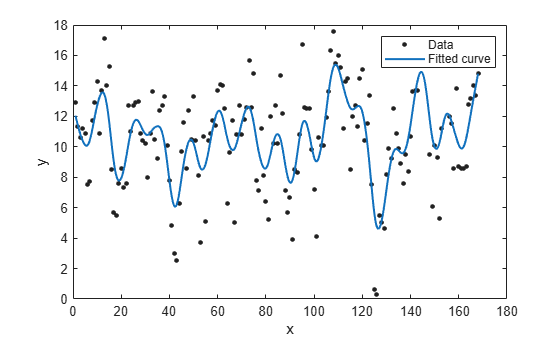Specify Smoothing Parameter using `fitoptions`

Alternatively, use `fitoptions` to specify a smoothing parameter before fitting.

```options = fitoptions('Method','Smooth','SmoothingParam',0.07); [f,gof,out] = fit(month,pressure,'smooth',options); out.p```
```ans = 0.0700 ```

For an alternative to `'smoothingspline'`, you can use the csaps cubic smoothing spline function or other spline functions that allow greater control over what you can create. See About Splines in Curve Fitting Toolbox.

### Example: Nonparametric Fitting with Cubic and Smoothing Splines

This example fits some data using a cubic spline interpolant and several smoothing splines.

1. Create the variables in your workspace:

```x = (4*pi)*[0 1 rand(1,25)]; y = sin(x) + .2*(rand(size(x))-.5); ```

2. Open the Curve Fitting app by entering:

`cftool`

3. Select `x` and `y` from the X data and Y data lists.

The Curve Fitting app fits and plots the data.

4. Fit the data with a cubic spline interpolant by selecting Interpolant fit type and the Method `Cubic`.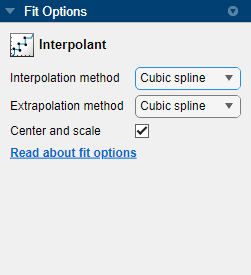The Curve Fitting app fits and plots the cubic spline interpolant.

5. Enter the Fit name `cubicsp`.

6. View the Results pane. Goodness-of-fit statistics such as RMSE are not defined (shown as `NaN`) for interpolants.A cubic spline interpolation is defined as a piecewise polynomial that results in a structure of coefficients (`p`). The number of “pieces” in the structure is one less than the number of fitted data points, and the number of coefficients for each piece is four because the polynomial degree is three. You can examine the coefficient structure `p` if you export your fit to the workspace (e.g., enter `fitname.p`). For information on the structure of coefficients, see Constructing and Working with ppform Splines.

7. Create another fit to compare. Right-click your fit in the Table of Fits and select Duplicate ‘cubicsp’.

8. Fit the data with a smoothing spline by selecting Smoothing Spline.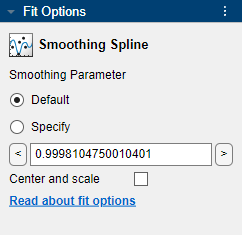The level of smoothness is given by the Smoothing Parameter. The default smoothing parameter value depends on the data set, and is automatically calculated by the toolbox.

For this data set, the default smoothing parameter is close to 1, indicating that the smoothing spline is nearly cubic and comes very close to passing through each data point.

9. Name the default smoothing parameter fit `Smooth1`. If you do not like the level of smoothing produced by the default smoothing parameter, you can specify any value from 0 to 1. 0 produces a linear polynomial fit, while 1 produces a piecewise cubic polynomial fit that passes through all the data points.

The numerical results for the smoothing spline fit are shown here.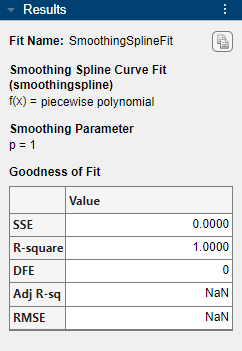10. For comparison purposes, create another smoothing spline fit. Right-click your fit in the Table of Fits and select Duplicate ‘smooth1’. Change the smoothing parameter to `0.5` and name the fit `Smooth2`.

11. Compare the plots for your three fits. Explore the fit behavior beyond the data limits by increasing the default abscissa scale. You change the axes limits with Tools > Axes Limit Control menu item.

Note

Your results depend on random start points and may vary from those described.

Note that the default smoothing parameter produces a curve that is smoother than the interpolant, but is a good fit to the data. In this case, decreasing the smoothing parameter from the default value produces a curve that is smoother still, but is not a good fit to the data. As the smoothing parameter increases beyond the default value, the associated curve approaches the cubic spline interpolant. The cubic spline and default smoothing spline are similar for interior points, but diverge at the end points.